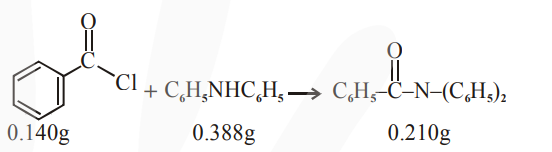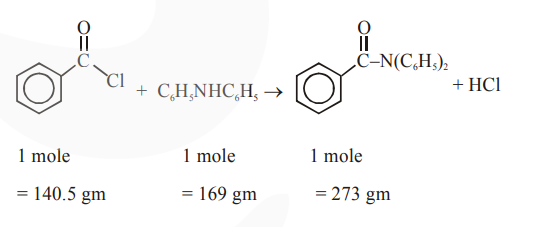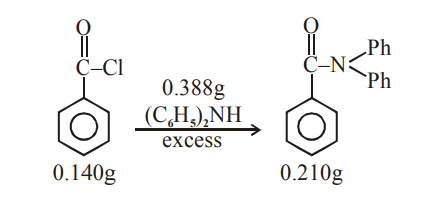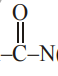Deepak Scored 45->99%ile with Bounce Back Crack Course. You can do it too!

# Solve this following

Question:Consider the above reaction. The percentage yield of amide product is (Round off to the Nearest Integer).

(Given : Atomic mass : $\mathrm{C}: 12.0 \mathrm{u}, \mathrm{H}: 1.0 \mathrm{u}$, $\mathrm{N}: 14.0 \mathrm{u}, \mathrm{O}: 16.0 \mathrm{u}, \mathrm{Cl}: 35.5 \mathrm{u})$

Solution:$\therefore 0.140 \mathrm{gm} \quad \frac{169}{140.5} \times 0.140$

$\begin{array}{ll}\text { L.R. } & =0.168 \mathrm{gm}<0.388 \mathrm{gm}\end{array}$

excess

$\therefore$ Theoretical amount of given product formed

$=\frac{273}{140.5} \times 0.140=0.272 \mathrm{gm}$

But its actual amount formed is $0.210 \mathrm{gm}$. Hence, the percentage yield of product.

$=\frac{0.210}{0.272} \times 100=77.20 \approx 77$Mole of $\mathrm{Ph}-\mathrm{CoCl}=\frac{0.140}{140}=10^{-3} \mathrm{~mol}$

Mole of $\mathrm{Ph}$$(\mathrm{Ph})_{2}$, that should be obtained

by mol-mol analysis $=10^{-3} \mathrm{~mol}$.

Theoritical mass of product $=10^{-3} \times 273=$ $273 \times 10^{-3} \mathrm{~g}$

Observed mass of product $=210 \times 10^{-3} \mathrm{~g}$

$\%$ yield of product $=\frac{210 \times 10^{-3}}{273 \times 10^{-3}} \times 100=76.9 \%=77$